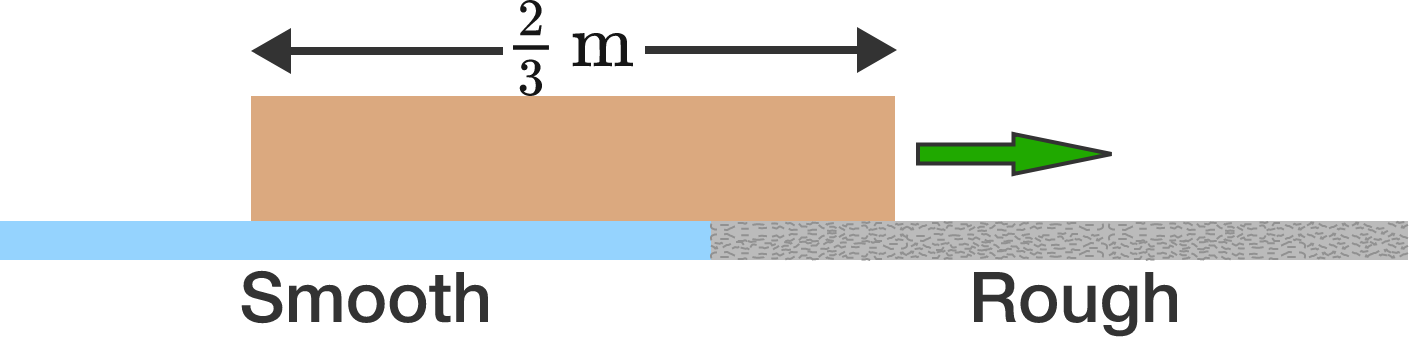# Box on Two SurfacesA rectangular box of length $\frac{2}{3} \text{m}$ is initially traveling at $2 \text{ m/s},$ with its entire length over the smooth (perfectly frictionless) blue surface (shown above). The box gradually moves onto a rough surface (gray) and stops the instant that its entire length is positioned within the rough region.

Determine the coefficient of kinetic friction.


Details and Assumptions:

• $g = 10\text{ m/s}^2.$
• The pressure at the bottom of the box is always uniform over its area.
×